Test: Syntax Directed Translation- 2

# Test: Syntax Directed Translation- 2

Test Description

## 7 Questions MCQ Test GATE Computer Science Engineering(CSE) 2023 Mock Test Series | Test: Syntax Directed Translation- 2

Test: Syntax Directed Translation- 2 for Computer Science Engineering (CSE) 2023 is part of GATE Computer Science Engineering(CSE) 2023 Mock Test Series preparation. The Test: Syntax Directed Translation- 2 questions and answers have been prepared according to the Computer Science Engineering (CSE) exam syllabus.The Test: Syntax Directed Translation- 2 MCQs are made for Computer Science Engineering (CSE) 2023 Exam. Find important definitions, questions, notes, meanings, examples, exercises, MCQs and online tests for Test: Syntax Directed Translation- 2 below.
Solutions of Test: Syntax Directed Translation- 2 questions in English are available as part of our GATE Computer Science Engineering(CSE) 2023 Mock Test Series for Computer Science Engineering (CSE) & Test: Syntax Directed Translation- 2 solutions in Hindi for GATE Computer Science Engineering(CSE) 2023 Mock Test Series course. Download more important topics, notes, lectures and mock test series for Computer Science Engineering (CSE) Exam by signing up for free. Attempt Test: Syntax Directed Translation- 2 | 7 questions in 21 minutes | Mock test for Computer Science Engineering (CSE) preparation | Free important questions MCQ to study GATE Computer Science Engineering(CSE) 2023 Mock Test Series for Computer Science Engineering (CSE) Exam | Download free PDF with solutions
 1 Crore+ students have signed up on EduRev. Have you?
Test: Syntax Directed Translation- 2 - Question 1

### Consider the following two statements: P: Every regular grammar is LL(1) Q: Every regular set has a LR(1) grammar Which of the following is TRUE?

Detailed Solution for Test: Syntax Directed Translation- 2 - Question 1

P:- This is false.
Every regular language is LL(1) meaning we have a LL(1) grammar for it. But we can not say same about every Regular Grammar. For example, every regular language can be represented by Left & Right Linear Grammar, where Left Linear Grammar is not LL(1), Right linear is.

Example aa* we can represent this as S->Sa|a which is not LL(1) ,but S->a|aS is LL(1).

Q:- This is true because of every LL(1) is LR(1).

All regular sets have Right recursive grammar, which is LL(1) & Every LL(1) is LR(1).
We can also say that LR(1) accepts DCFL & Regular languages are subset of DCFL.

Test: Syntax Directed Translation- 2 - Question 2

### Consider the CFG with {S,A,B) as the non-terminal alphabet, {a,b) as the terminal alphabet, S as the start symbol and the following set of production rules: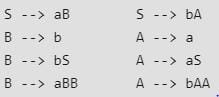Which of the following strings is generated by the grammar?

Detailed Solution for Test: Syntax Directed Translation- 2 - Question 2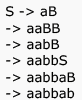Test: Syntax Directed Translation- 2 - Question 3

### Consider the CFG with {S,A,B) as the non-terminal alphabet, {a,b) as the terminal alphabet, S as the start symbol and the following set of production rules: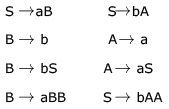For the string aabbab , how many derivation trees are there?

Detailed Solution for Test: Syntax Directed Translation- 2 - Question 3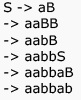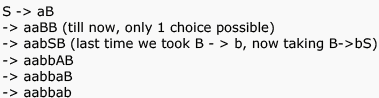Test: Syntax Directed Translation- 2 - Question 4

Which of the following statements are true?

I. Every left-recursive grammar can be converted to a right-recursive grammar and vice-versa

II. All ∈ -productions can be removed from any context-free grammar by suitable transformations

III. The language generated by a context-free grammar all of whose productions are of the form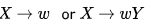(where ω ,  is a string of terminals and  is a non-terminal), is always regular

IV. The derivation trees of strings generated by a context-free grammar in Chomsky Normal Form are always binary trees

Detailed Solution for Test: Syntax Directed Translation- 2 - Question 4

Statement 1 is true: Using GNF we can convert Left recursive grammar to right recursive and by using reversal of CFG and GNF we can convert right recursive to left recursive.

Statement 2 is false: because if ∊ is in the language then we can't remove ∊ production from Start symbol. (For example L = a*)

Statement 3 is true because right linear grammar generates regular set

Statement 4 is true, only two non-terminals are there in each production in CNF. So it always form a binary tree.

Test: Syntax Directed Translation- 2 - Question 5

The grammar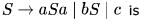Detailed Solution for Test: Syntax Directed Translation- 2 - Question 5

For LL(1) take First(S). and do intersection between the result. if intersection is Phi then LL(1) else not.

Making a parsing table and checking if there are two or more entries under any terminal. If yes then neither LL(1) nor LR(1).

Test: Syntax Directed Translation- 2 - Question 6

Consider an ambiguous grammar G and its disambiguated version D. Let the language recognized by the two grammars be denoted by L(G) and L(D) respectively. Which one of the following is true?

Detailed Solution for Test: Syntax Directed Translation- 2 - Question 6

L (D) = L (G) , Both must represent same language .Also  if we are converting  a grammar from Ambiguous to UnAmbiguous form , we must  ensure that our new new grammar represents the same language as previous grammar.

For ex G1: S->Sa/aS/a; {Ambiguous (2 parse trees for string 'aa')}

G1':S->aS/a;{Unambiguous}

Both represents language  represented by regular expression: a^+

Test: Syntax Directed Translation- 2 - Question 7

Consider these three grammars.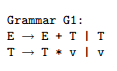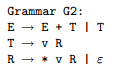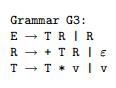Which of the following statements is not true?

Detailed Solution for Test: Syntax Directed Translation- 2 - Question 7

In G1, T can take the form of v * v * v * v * v ....
In G2, R can take the form * v * v * v ...,  so T can take the form of v * v * v * v ....

Thus, in both G1 and G2, E can take the form v * v * v + v * v * v * v + v * v ....

These are the algebraic expressions involving only addition and multiplication.

In G3, T can take the form v * v * v * v, so R can take the form + v * v + v * v. Thus, through the production E ! T R, E can take the form of any arithmetic expression.

However, E can also take the form of non-arithmetic expressions,

as with E --> R --> + T R --> + v R --> + v + T R ---> + v + v R --> + v + v.

In addition, G3 can generate ε , which G1 and G2 cannot.

Thus, while G1 and G2 generate the same language,

G3 generates a somewhat larger language. Incidentally, G1 demonstrates left-recursion because it contains productions of the form X --> X  α ( in addition to non-problematic productions of the form   X --> β ).

Left recursion can be eliminated by introducing a helper non-terminal R and changing the grammar to read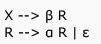## GATE Computer Science Engineering(CSE) 2023 Mock Test Series

149 docs|215 tests
 Use Code STAYHOME200 and get INR 200 additional OFF Use Coupon Code
Information about Test: Syntax Directed Translation- 2 Page
In this test you can find the Exam questions for Test: Syntax Directed Translation- 2 solved & explained in the simplest way possible. Besides giving Questions and answers for Test: Syntax Directed Translation- 2, EduRev gives you an ample number of Online tests for practice

## GATE Computer Science Engineering(CSE) 2023 Mock Test Series

149 docs|215 tests